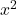## write a quadratic equation with the roots 1/2 and -5

Question

write a quadratic equation with the roots 1/2 and -5

in progress 0
2 months 2021-08-04T19:37:32+00:00 2 Answers 0 views 0

2+ 9x – 5 = 0

Step-by-step explanation:

// Use the Vijet’s theorem,

// note that the kofficient before= 1

Sum of the roots is:

1/2 + (-5) = – 4,5

Product of the roots is:

0,5 * (-5) = – 2,5– (sum of the roots) x + product of the roots  =  0– (-4,5) * x + (-2,5) = 0+ 4,5x -2,5 = 0

// multiply both sides by 2

2+ 9x – 5 = 0

Make me the brainliest 😉

2. x = 1/2    or    x = -5

2x – 1 = 0     or     x + 5 = 0

(2x-1)(x+5) = 0

2x² + 10x – x – 5 = 0

2x² + 9x – 5 = 0

Hope it helps!